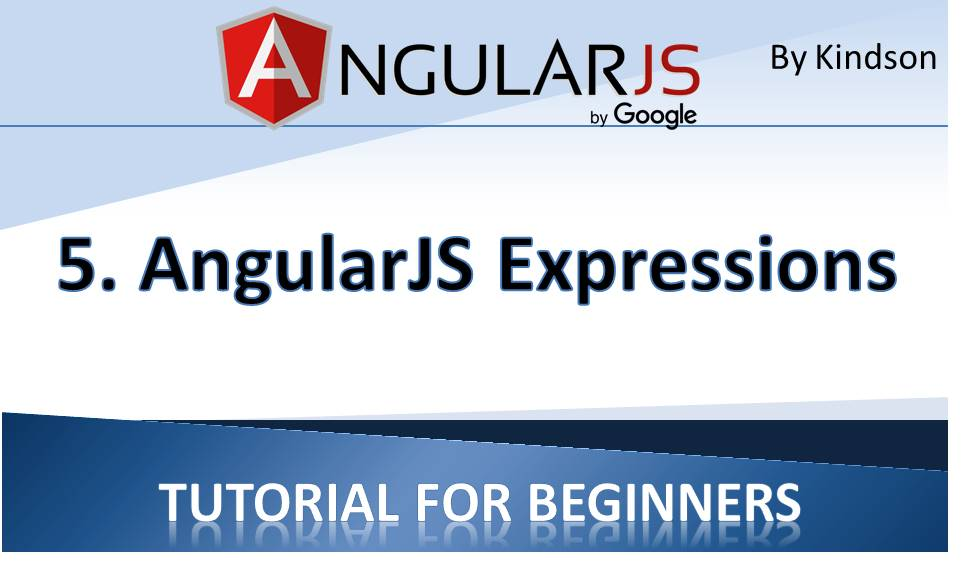# AngularJS Tutorial for Beginners 5 – AngularJS ExpressionsThis lesson would be brief and contains a simple exercise, make sure you do it.

AngularJS Expressions are expression written inside a normal html file but are interpreted by AngularJS. AngularJS expressions are enclosed in double curly braces like this:

{{ expression }}
They can also be written inside a directive using an assignment operator, for example:
ng-model = {{ expression }}
AngularJS will evalulate the expression, and output the result exactly where the expression is written in the html file.

AngularJS expression can contain strings, numbers, operators and other variables.

Examples of AngularJS expressions are:

{{ 88 / 4 }}
{{ firstname + “, ” + lastname }}
{{ (2 * 5)/10 }}

Exercise
This exercise follows from Lesson 4.
Create a new file in the same folder you placed Lesson 4 and save it as Lesson5.html.

Place the following code in this file and take some time to go through the code to understand how the angularJS expressions are formed. Also take not of the use of arrays.

`<!doctype html>`

<html ng-app>
<title>AngularJS Tutorial for Beginners</title>

<script src=“angular.min.js”></script>
<script src=“Scrips.js”></script>

<body>
<h1>AngularJS Expression – Lesson 5</h1>

<div ng-app = “” ng-init = “quantity = 1; price = 30; student = {firstname:’Kindson’,lastname:’Munonye’,regno:242}; code = ‘CS101’; scores = [80,90,75,73,60]”>
<p>Student {{student.firstname + ” ” + student.lastname}}!</p>
<p>Code: {{code}}</p>
<p>Cost on Books : {{price * quantity}} USD</p>
<p>Reg No: {{student.regno}}</p>
<p>Scores(English): {{scores}}</p>
</div>
</body>
</html>

Note on Arrays
Arrays are used in the same way in AngularJS as in JavaScript. In the example, a one-dimensional array called scores has been initialized:

`scores = [80,90,75,73,60]`

To refer to a single element of the array you can use the index of the array and remember the the first element of the array has an index of 0.
So scores refers to 80
scores refers to 90 and so on.

I hope that by now you are very clear with AngularJS expressions. If you have any challenge, do leave a comment below. In Lesson 6, we would then examine angularJS controllers in more details.